# SSAT Upper Level Math : Rational Numbers

## Example Questions

1 2 36 37 38 39 40 41 42 44 Next →

### Example Question #431 : Rational Numbers

Find the sum of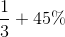.  Express the final answer as a fraction.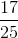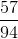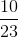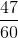Explanation:

Convert the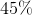into fraction form by dividing this by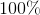.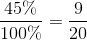First find the common denominator by multiplying the current denominators together.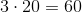Now convert each fraction into an equivalent fraction with the new, common denominator.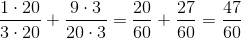### Example Question #432 : Rational Numbers

What percentage of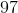is? Round to the nearest hundredth.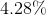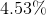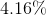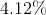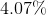Explanation:

Set up a mathematical equation such that the percentage of the number is divided by 100. Solve for the unknown value.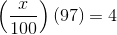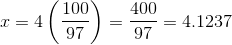The correct answer is.

### Example Question #433 : Rational Numbers

Each of a set of balls is marked with a letter of the English alphabet. Each vowel is represented by five balls; each consonant, three. What percent of the balls are marked with vowels? (Nearest whole percent)

Note: For purposes of this problem, "Y" is considered a consonant.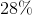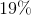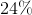Explanation:

There are five vowels, each represented by five balls; this is a total of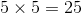balls with vowels.

There are twenty-one consonants, each represented by three balls; this is a total of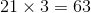balls with consonants.

There are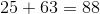balls total of which 25 out of 88 are marked with vowels. This represents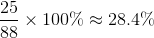of the balls, so 28% is the correct choice.

1 2 36 37 38 39 40 41 42 44 Next →

### All SSAT Upper Level Math Resources